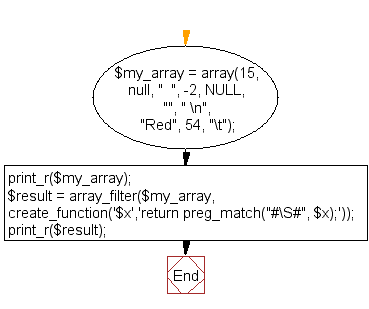﻿ PHP Array Exercise: Remove all white spaces in an array - w3resource# PHP Array Exercises : Remove all white spaces in an array

## PHP Array: Exercise-54 with Solution

Write a PHP script to remove all white spaces in an array.

Sample Solution:

PHP Code:

``````<?php
\$my_array = array(15, null, "  ", -2, NULL, "", " \n", "Red", 54, "\t");
print_r(\$my_array);
\$result = array_filter(\$my_array, create_function('\$x','return preg_match("#\S#", \$x);'));
print_r(\$result);
?>
```
```

Sample Output:

```Array
(
 => 15
 =>
 =>
 => -2
 =>
 =>
 =>

 => Red
 => 54
 =>
)
Array
(
 => 15
 => -2
 => Red
 => 54
)
```

Flowchart:PHP Code Editor:

What is the difficulty level of this exercise?

﻿

## PHP: Tips of the Day

PHP: Extract numbers from a string

```\$str = 'In My Cart : 11 12 items';
preg_match_all('!\d+!', \$str, \$matches);
print_r(\$matches);
```